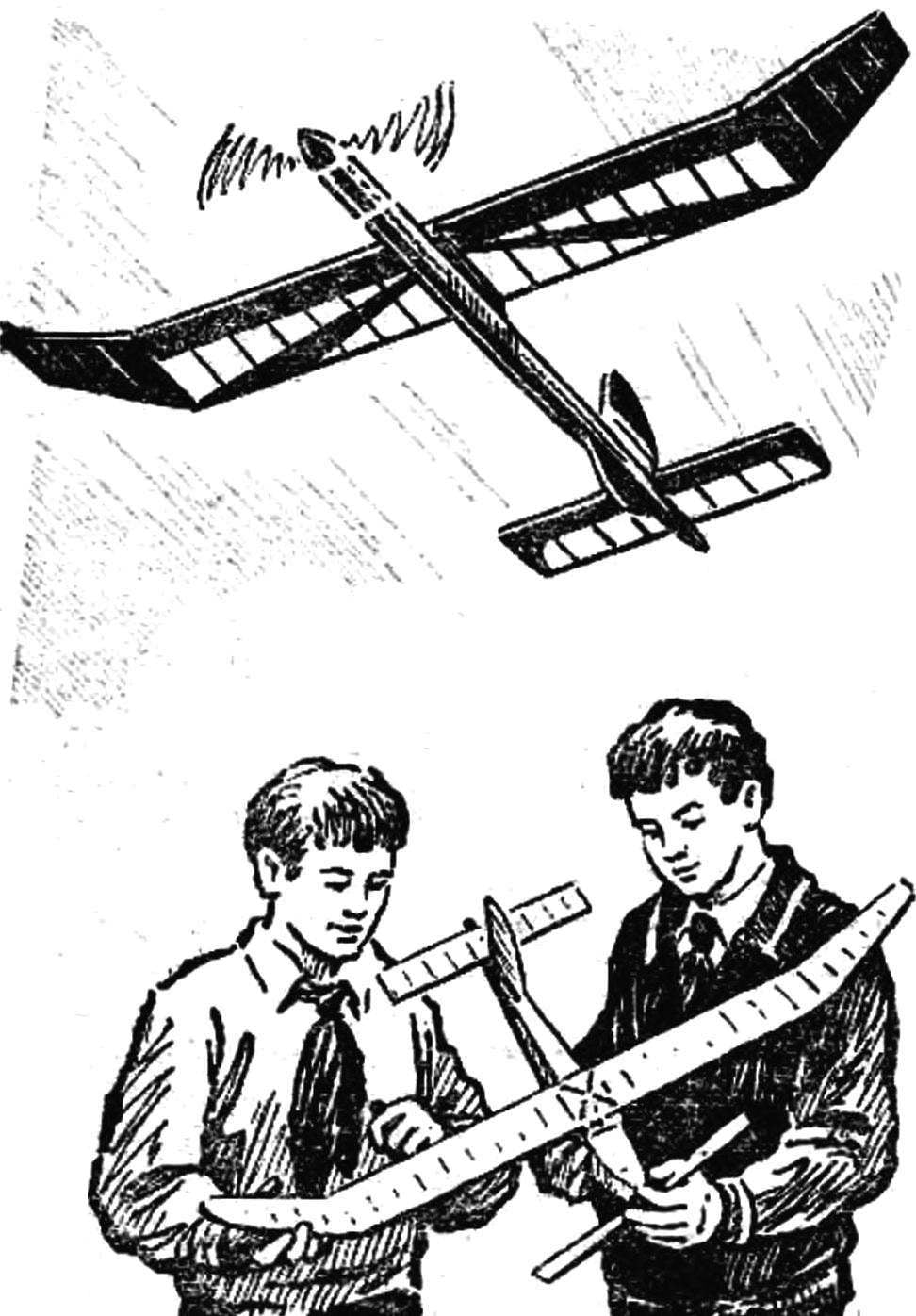# THE SECRETS OF THE RUBBER MOTOR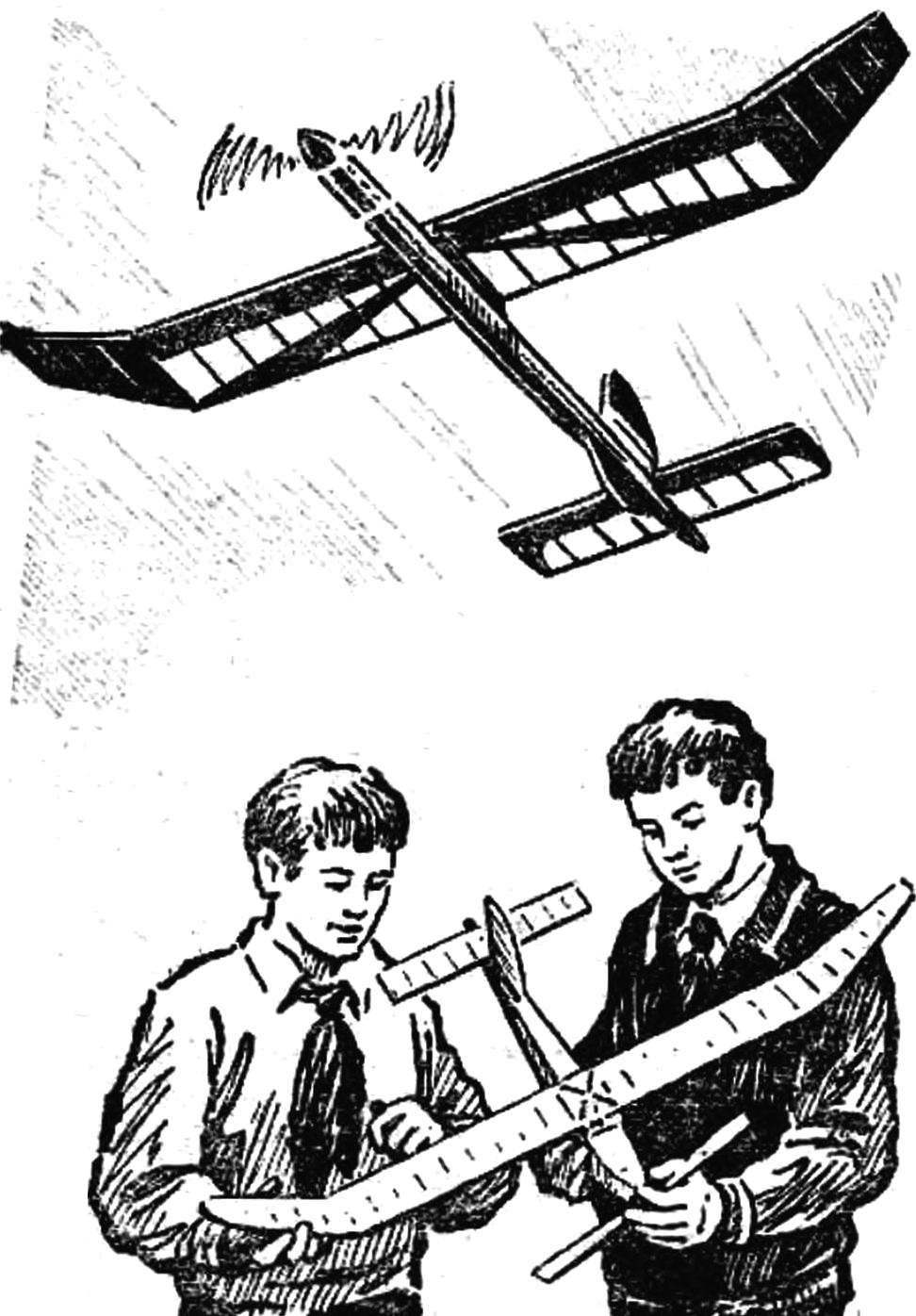For years, the rubber motor due to its ease of use and low cost were the main engine modelers. But now, with the abundance of micro-motors boat and car with rubber motor find its ardent supporters, as evidenced, in particular, and editorial mail. Among your questions, dear readers, the most frequent two. So T zavgorodnego from Volgograd, E. Shcherbina from Semipalatinsk, A. Zhdanov of Moscow and other interested in how to calculate the number of turns of the rubber motor to maximize its energy, and what is its maximum energy efficiency.

The rubber motor is powered by the internal energy of the stretched rubber threads. To increase the length they can pretty much 6-7 times or more, and distinguishable varieties are stretched in different ways. There are several formulas that can be used by modelers in calculating the rubber motor. One of them can determine the relative elongation λ (lambda) is the ratio of the increment of length to the original. In a formula it is written like this: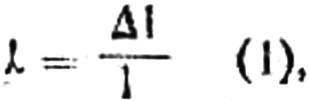where 1 is the length of the rubber in the free state, and Δ1 is the increment of length.

Stretching rubber thread, we spend a certain amount of energy, but get back only 65— 67%. Loss Vizcaya costs of internal friction in the rubber. Consequently, the coefficient of performance (COP) of the rubber motor can not be greater than 0,65—0,67.
To accumulate in the rubber motor more energy needed, saving a lot of rubber to increase spin. And it will be the smaller, the thicker the rubber motor. This contradiction is solved by the preparation of the rubber motor of several individual threads. As in the promotion of the rubber motor power loss is called as internal friction and friction between the individual filaments, the practical speed is always less than that which would be obtained by calculations. Предла1аем an approximate formula for calculating speed: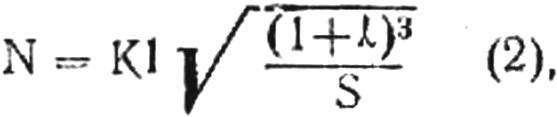here: N is the maximum factory rubber motor in rpm,

E — the initial length of the rubber motor in centimeters,

λ is the maximum elongation according to the characteristics of the rubber or experimentally derived,

S — the total cross section of the strands of the rubber motor in cm2,

It is some experimental factor.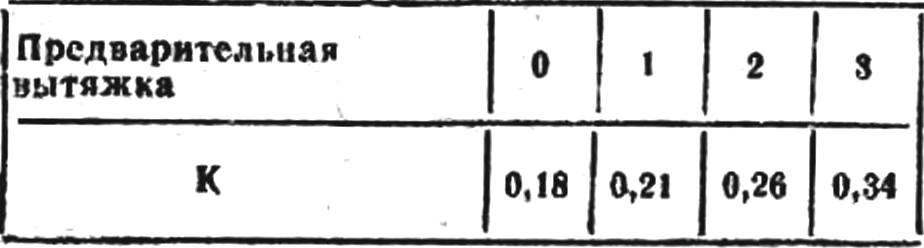It should be said that the accuracy of the calculation by this formula will increase if the rubber motor before the factory pre-pull. Experiments showed that with increasing pre-exhaust the number of revolutions increases, which takes into account the introduction of the experimental coefficient K in formula 2. Such an impact on the plant due to, apparently, the fact that the distribution of friction between the fibers, and particularly between the threads of the rubber motor is changed.

The values of experimental coefficient K, depending on the preliminary drawing is given in the table.

To reduce friction between the rods, the rubber motor is usually smeared with castor oil or glycerin (and this increases its durability). If you take useful energy of the rubber motor for 100%, for screwing without pre-exhaust we will lose 30-35% of friction and brushing it and applying pre-extract 1 to 3 times, it is possible to reduce losses to 5%.

A. you KNOW